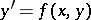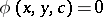Singular solution

of an ordinary differential equation

A solution at every point of which the uniqueness of the solution of the Cauchy problem for this equation is violated. For example, for an equation of the first order(*)

with a continuous right-hand side which has a finite or infinite partial derivative everywhere with respect to, a singular solution can only lie in the setA curveis a singular solution of (*) ifis an integral curve of the equation (*) and if at least one more integral curve of (*) passes through every point of. Let equation (*) have a general integralin a domain; if this family of curves has an envelope, then this is a singular solution of equation (*). For a differential equationa singular solution is found by examining the discriminant curve.